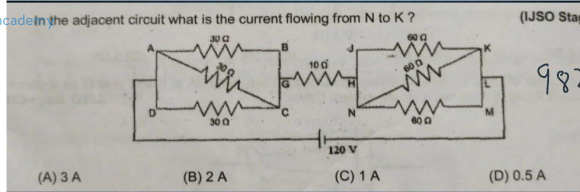"
">

# What is the current flowing from N to K in the circuit given below?"

Solution:

The equivalent resistance of the first parallel combination of three 30-ohm resistances

1/R = 1/30 + 1/30 + 1/30 = 3/30

R = 30/3 = 10 ohm

The equivalent resistance of the second parallel combination of three 60-ohm resistances

1/R = 1/60 + 1/60 + 1/60 = 3/60

R = 60/3 = 20 ohm

There is a 10-ohm resistance in series between these two parallel combinations

So the equivalent resistance of the series circuit of 10, 10 and 20 ohms

is 10-ohm + 10-ohm + 20-ohm = 40-ohm

The current in circuit = 120 V/40-ohm = 3 A

This current gets divided between three 60 ohms equally

The current flowing from N to K = 3/3 = 1 ampere

So option (C) 1A is CORRECT

Updated on: 10-Oct-2022

48 Views